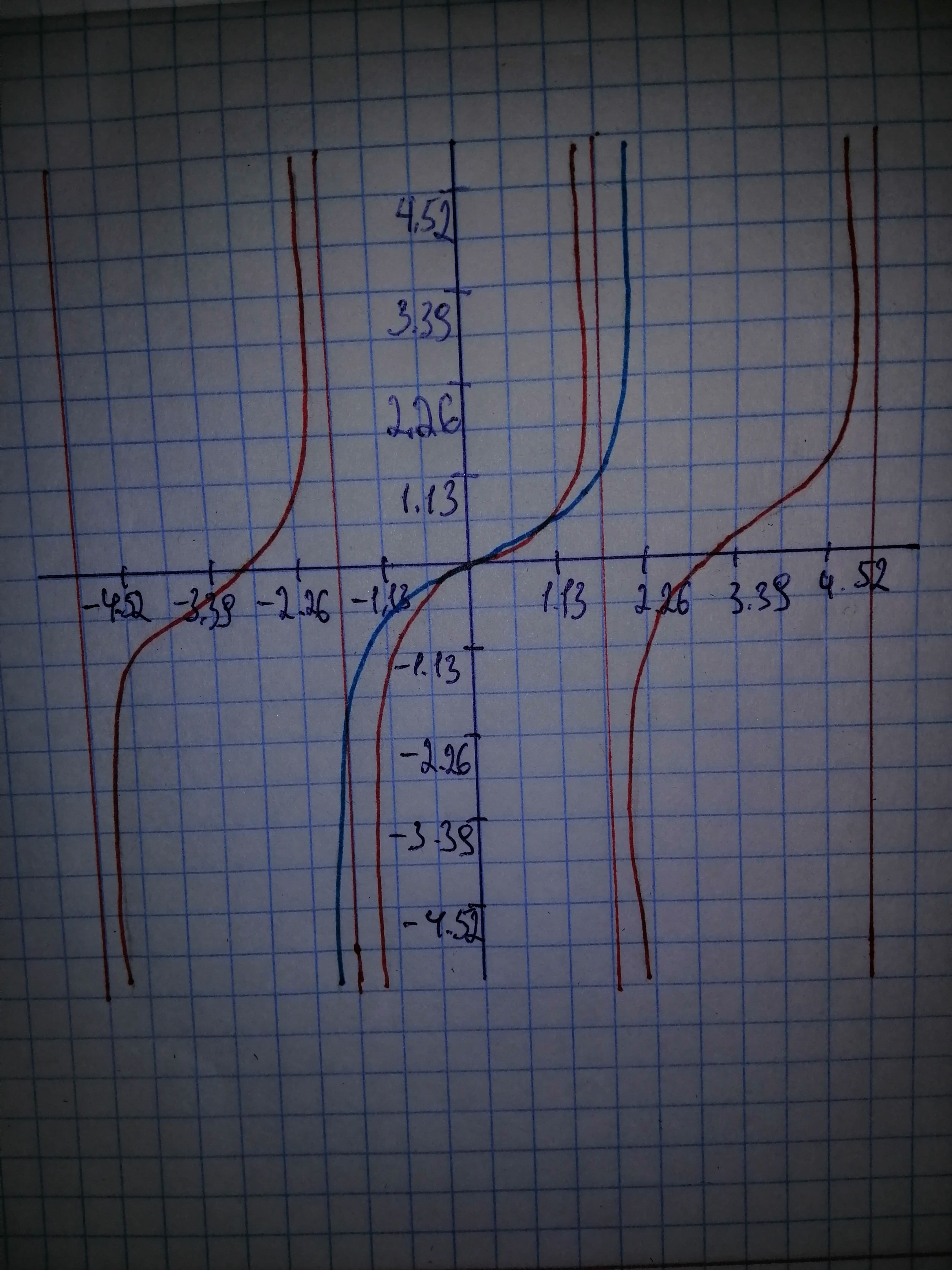# Using calculus, it can be shown that the tangent function can be approximated by the polynomial tan x approx x + frac{2x^{3}}{3!} + frac{16x^{5}}{5!}cistG 2021-02-15 Answered
Using calculus, it can be shown that the tangent function can be approximated by the polynomial where x is in radians. Use a graphing utility to graph the tangent function and its polynomial approximation in the same viewing window. How do the graphs.
You can still ask an expert for help

• Questions are typically answered in as fast as 30 minutes

Solve your problem for the price of one coffee

• Math expert for every subject
• Pay only if we can solve itodgovoreh

The red graph is tangent and the blue graph is approximation. The graphs aresimilar close to when $x=0$.